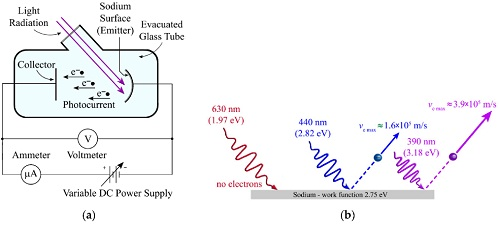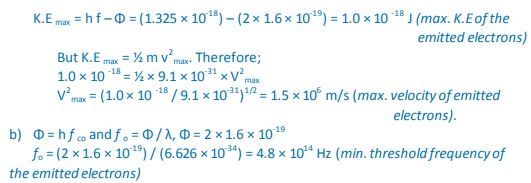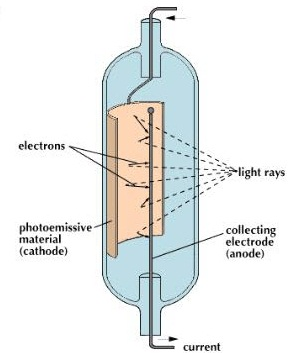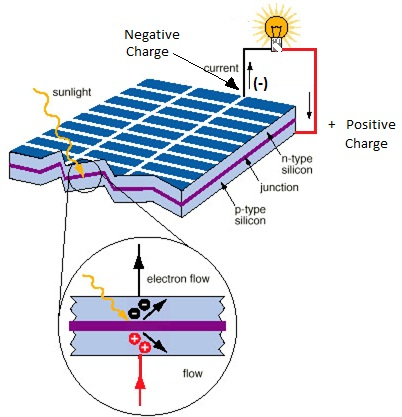# Photoelectric Effect

### Guest Account

Attempt Form Four Physics Questions
Guest Account

# Photoelectric Effect

- Photoelectric effect was discovered by Heinrich Hertzin 1887.
Photoelectric effect is a phenomenon in which electrons are emitted from the surface of a substance when certain electromagnetic radiation falls on it.
- Metal surfaces require ultra-violet radiation while caesium oxide needs a visible light i.e. optical spectrum (sunlight).## Work Function

- A minimum amount of work is needed to remove an electron from its energy level so as to overcome the forces binding it to the surface.
- This work is known as the work function with units of electron volts (eV).
- One electron volt is the work done when one electron is transferred between points with a potential difference of one volt; that is:

1 eV = 1 electron × 1 volt
1 eV = 1.6 × 10,-19 × 1 volt
1 eV = 1.6× 10-19 Joules (J)

#### Threshold frequency

This is the minimum frequency of the radiation that will cause a photoelectric effect on a certain surface. The higher the work function, the higher the threshold frequency.

#### Factors affecting the photoelectric effect

1. Intensity of the incident radiation– the rate of emission of photoelectrons is directly proportional to the intensity of incident radiation.
2. Work function of the surface– photoelectrons are emitted at different velocities with the maximum being processed by the ones at the surface.
3. Frequency of the incident radiation– the cut-off potential for each surface is directly proportional to the frequency of the incident radiation.

## Planck's constant

- When a bunch of oscillating atoms and the energy of each oscillating atom is quantified i.e. it could only take discrete values. Max Planck's predicted the energy of an oscillating atom to be:
E = n h f, where n – integer, f – frequency of the source, h – Planck's constant which has a value of 6.63 × 10-34 Js.

#### Quantum theory of light

Planck's published his quantum hypothesis in 1901 which assumes that the transfer of energy between light radiation and matter occurs in discrete units or packets. Einstein proposed that light is made up of packets of energy called photons which have no mass but they have momentum and energy given by:
E = h f
The number of photons per unit area of the cross-section of a beam of light is proportional to its intensity. However the energy of a photon is proportional to its frequency and not the intensity of the light.

## Einstein's Photoelectric Equation

As an electron escapes, energy equivalent to the work function of the emitter substance is 'given up'.
So the photon energy must be greater than or equal to Φ. If the ‘h f' is greater than Φ then the electron acquires some kinetic energy after leaving the surface. The maximum kinetic energy of the ejected photoelectron is given by;
K.E max = ½ m v2
max = h f – Φ ………………(i), where m v2
max = maximum velocity and mass.
This is the Einstein's photoelectric equation.
If the photon energy is just equivalent to work function then, m v2
max = 0, at this juncture the electron
will not be able to move hence no photoelectric current, giving rise to a condition known as cut-off frequency, h fco = Φ………………. (ii)
Also the p.d required to stop the fastest photoelectron is the cut-off potential, V cowhich is given by E = e V co electron volts, but this energy is the maximum kinetic energy of the photoelectrons and therefore, ½ m v2
max = e V co ………….. (iii).
Combining equations (i), (ii) and (iii), we can write Einstein's photoelectric equation as, e V co = h f – h fco ………………….. (iv)

NOTE: -- Equations (i) and (iv) are quite useful in solving problems involving photoelectric effect.

Examples
1. The cut-off wavelength for a certain material is 3.310 × 10-7 m. What is the cut-off frequency for the material?
Solution
Speed of light ‘c' = 3.0 × 108 m/s. Since f = c / λ, then
f = 3.0 × 108/ 3.310 × 10-7
= 9.06 × 1014 Hz.

2. The work function of tungsten is 4.52 e V. Find the cut-off potential for photoelectrons when a tungsten surface is illuminated with radiation of wavelength 2.50 × 10-7 m. (Planck's constant, h = 6.62 × 10-34 Js).
Solution
Frequency ‘f' = c / λ = 3.0 × 108/ 2.50 × 10-7
Energy of photon = h f = 6.62 × 10-34 × (3.0 × 108/ 2.50 × 10-7) × (1 / 1.6 × 10-19) = 4.97 eV.
Hence h fco = 4.52 e V. e V co = 4.97 e V - 4.52 e V = 0.45 e V = 7.2 × 10-20 J
V co = 7.2 × 10-20 / 1.6 × 10-19 = 0.45 e V.

3. The threshold frequency for lithium is 5.5 × 1014 Hz. Calculate the work function for lithium. (Take ‘h' = 6.626 × 10-34 Js)
Solution
Threshold frequency, f o = 5.5 × 1014 Hz, ‘h' = 6.626 × 10-34 Js
Φ = h f = 5.5 × 1014× 6.626 × 10-34 = 36.4 × 10-20

4. Sodium has a work function of 2.0 e V. Calculate
a) The maximum energy and velocity of the emitted electrons when sodium is Illuminated by a radiation of wavelength 150 nm.
b) Determine the least frequency of radiation by which electrons are emitted.
(Take ‘h' = 6.626 × 10-34 Js, e = 1.6 × 10-19, c = 3.0 × 108 m/s and mass of electron = 9.1 × 10-31 kg).
Solution
a) The energy of incident photon is given by h f = c / λ
= (6.626 × 10-34 × 3.0 × 108) / 1.50 × 10-9
= 1.325 × 10-18 J### Applications of photoelectric effect

1. Photo-emissive cells - they are made up of two electrodes enclosed in a glass bulb (evacuated or containing inert gas at low temperature). The cathode is a curved metal plate while the anode is normally a single metal rod)They are used mostly in controlling lifts (doors) and reproducing the sound track in a film. Photoconductive cells - some semi-conductors such as cadmium sulphide (cds) reduces their resistance when light is shone at them (photo resistors).Other devices such as photo-diodes and photo-transistors block current when the intensity of light increases.
- Photo-conductive cells are also known a slight dependent resistors (LDR) and are used in alarm circuits i.e. fire alarms, and also in cameras as exposure metres.
2. Photo-voltaic cell-They are used in electronic calculators, solar panels etc.